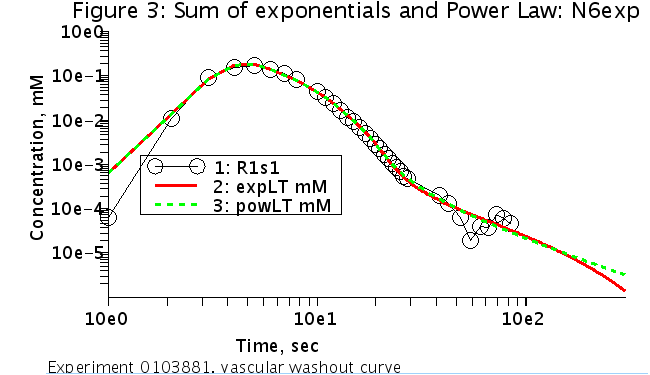# LongTailPowerLaw

Model number
0291

Fit the long tailed vascular output curves using N decaying exponentials where the ratio of the decay rates is constant and the ratio of the amplitudes is also constant.

## DescriptionFit the long tail of a vascular outflow curve from an indicator dilution
curve with the sum of N exponentials at tjoin matching both the function,
F, and the slope, S. It is assumed that S is negative.

N
-----
\
Tail(t) =        )   w(i)*exp( -k(i)*(t-tjoin) )
/
-----
i = 1
where w(i) = a*w(i+1), a>0;  k(i)=b*k(i=1), b>1.

If F is the value of the function and S is the slope at t=tjoin, then
the coefficients are given as
(N - i)
a        F (a - 1)
w(i) =  ------------------
N
a  - 1

(N - i)     N
b        S (a  - 1) (a b - 1)
k(i) = - -----------------------------
N
F (a - 1) ((a b)  - 1)

It can be shown that
N                  N
-----              -----
\                  \
F=   )   w(i)  and S=   )   -w(i)*k(i)
/                  /
-----              -----
i = 1              i = 1

The user can use optimization to  calculate values for a, b, and tjoin.
The limits on a and b are a>0, b>1.

POWER LAW

The long tail function can also be given as a power law expression of the form

/t - tjoin + tf\(-beta)
PowerLaw = A |--------------|
\     sec      /
where
/    F*beta  \beta
A = F |- ----------|
\  S*(1 sec) /
and
F beta
tf = - ------ .
S

For further information relating PowerLawly scaled models with power law models

## Equations

None.

The equations for this model may be viewed by running the JSim model applet and clicking on the Source tab at the bottom left of JSim's Run Time graphical user interface. The equations are written in JSim's Mathematical Modeling Language (MML). See the Introduction to MML and the MML Reference Manual. Additional documentation for MML can be found by using the search option at the Physiome home page.

References
 J.B. Bassingthwaighte and D.A. Beard. Fractal 15 O-labeled water
washout from the heart. Circ. Res. 77:1212-1221, 1995.

L.M. Schwartz, T.R. Bukowski, J.H. Revkin, and J.B. Bassingthwaighte.
Cardiac endothelial transport and metabolism of adenosine and inosine.
Am. J. Physiol. 227 (Heart Circ. Physiol. 46):1231-1251, 1999.

Key terms
Longtail
long tail
washout
albumin
optimization
fractal
exponential
indicator dilution
Acknowledgements

Please cite https://www.imagwiki.nibib.nih.gov/physiome in any publication for which this software is used and send one reprint to the address given below:
The National Simulation Resource, Director J. B. Bassingthwaighte, Department of Bioengineering, University of Washington, Seattle WA 98195-5061.

Model development and archiving support at https://www.imagwiki.nibib.nih.gov/physiome provided by the following grants: NIH U01HL122199 Analyzing the Cardiac Power Grid, 09/15/2015 - 05/31/2020, NIH/NIBIB BE08407 Software Integration, JSim and SBW 6/1/09-5/31/13; NIH/NHLBI T15 HL88516-01 Modeling for Heart, Lung and Blood: From Cell to Organ, 4/1/07-3/31/11; NSF BES-0506477 Adaptive Multi-Scale Model Simulation, 8/15/05-7/31/08; NIH/NHLBI R01 HL073598 Core 3: 3D Imaging and Computer Modeling of the Respiratory Tract, 9/1/04-8/31/09; as well as prior support from NIH/NCRR P41 RR01243 Simulation Resource in Circulatory Mass Transport and Exchange, 12/1/1980-11/30/01 and NIH/NIBIB R01 EB001973 JSim: A Simulation Analysis Platform, 3/1/02-2/28/07.